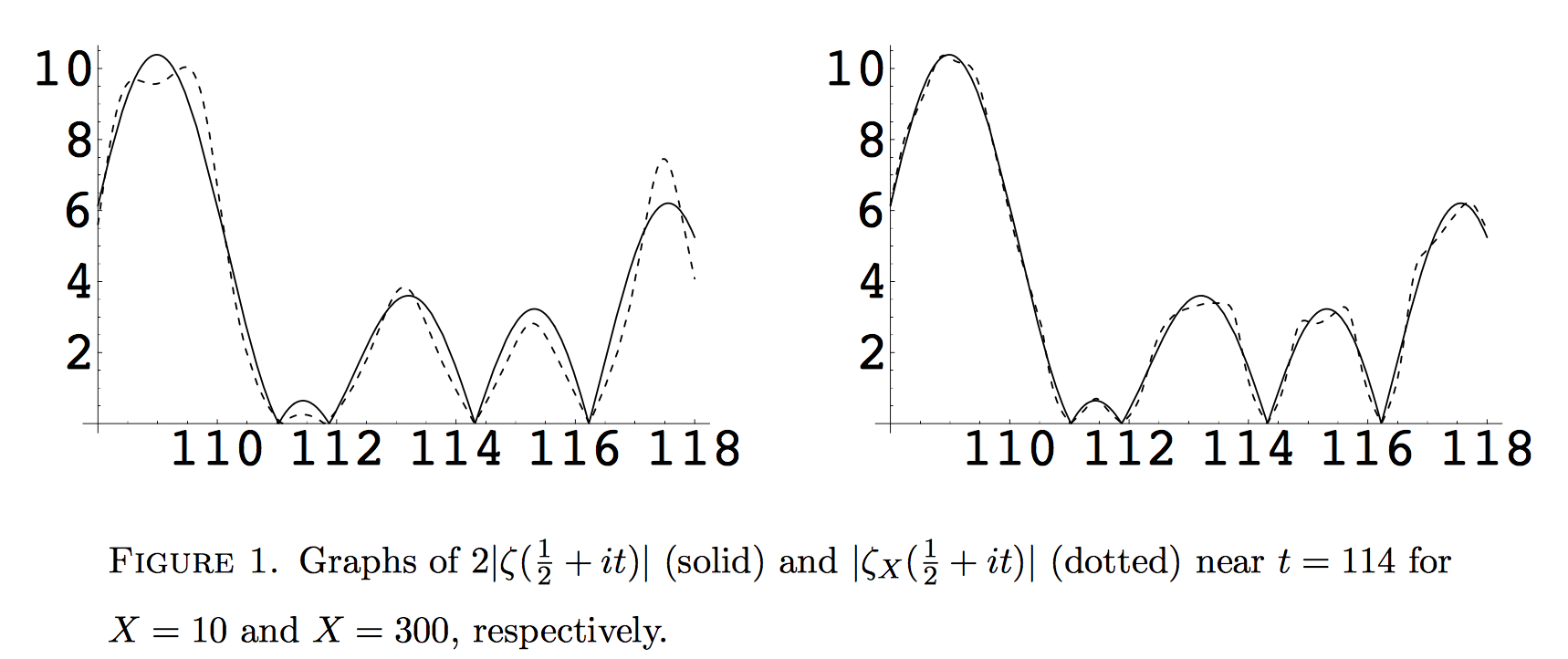# Euler product approximation of the Riemann zeta function

It is well-known that the Riemann zeta function can be approximated in the critical strip by a finite Dirichlet polynomial. More precisely, $$\zeta(\sigma+it) = \sum_{n \leq X} n^{-\sigma-it} + \frac{X^{1-\sigma-it}}{\sigma+it-1} + O ( X^{-\sigma})$$ uniformly for all $X$ satisfying $2 \pi X / C \geq |t|$, where $C$ is a fixed constant greater than 1. (This is Theorem 4.11 in Titchmarsh's book). In particular this holds for $\sigma = 1/2$.

Question: is there a similar (simple) approximation of zeta by a finite Euler product?

• PS: I want to avoid the large correction term of order $\approx X^{1-\sigma}$. This large correction term corresponds to the large number of summands in the Dirichlet polynomial. The idea is that an Euler product approximation would probably be "sparse" when written as a Dirichlet polynomial, thus not requiring this large correction term. – Kurisuto Asutora Mar 16 '17 at 8:05

## 1 Answer

See Finite Euler products and the Riemann Hypothesis by S. M. Gonek (2007):

We show that if the Riemann Hypothesis is true, then in a region containing most of the right-half of the critical strip, the Riemann zeta-function $$\zeta$$ is well approximated by short $$X$$-term truncations $$\zeta_X$$ of its Euler product. Conversely, if the approximation by products is good in this region, the zeta-function has at most finitely many zeros in it.This approximation also provides insight into why the zeroes on the critical line "repel", in the sense of level repulsion in the Gaussian Unitary Ensemble.

• Yes, I have seen this paper, but I cannot make much sense out of it. What's the role of the function Z in their paper? Is it large, is it small? – Kurisuto Asutora Mar 15 '17 at 9:22
• PS: I should note that I am looking for unconditional results (without assuming RH). – Kurisuto Asutora Mar 15 '17 at 9:24